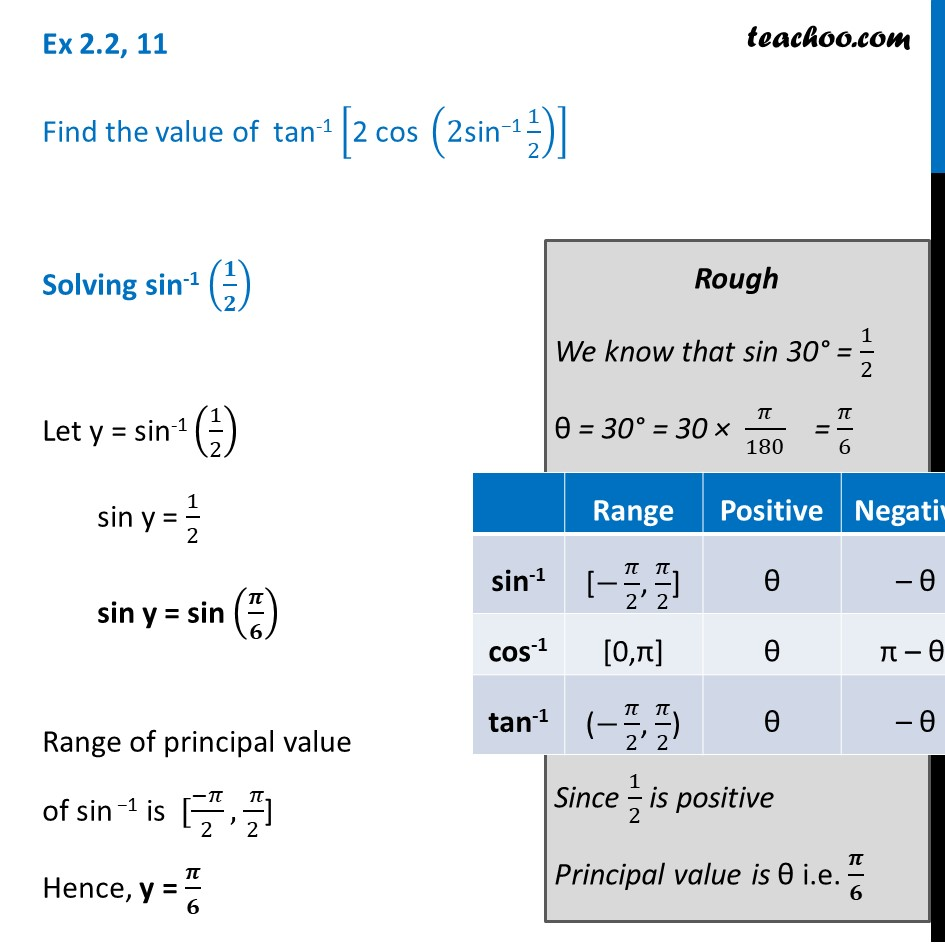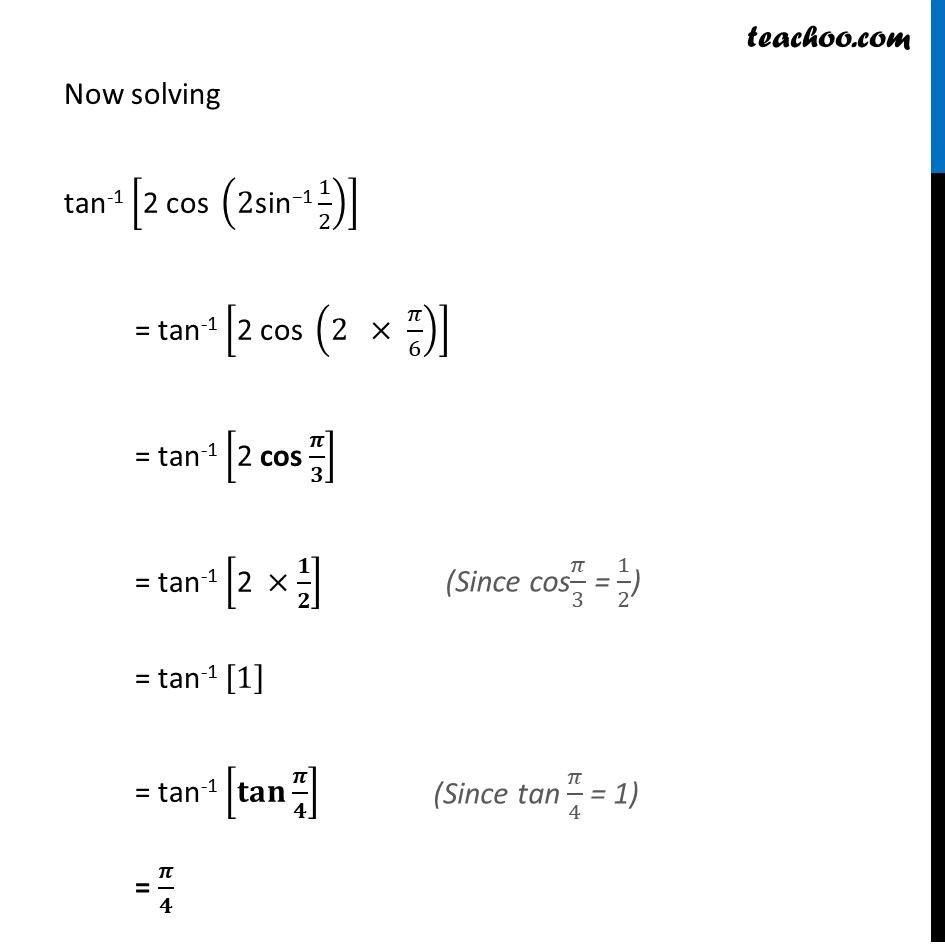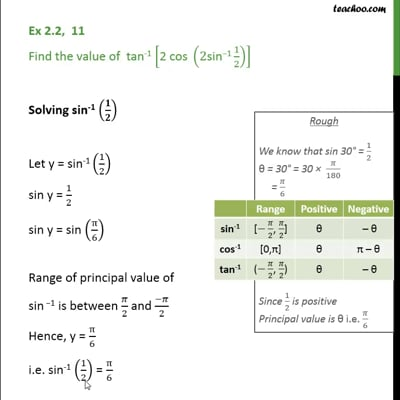Ex 2.2

Chapter 2 Class 12 Inverse Trigonometric Functions
Serial order wiseThis video is only available for Teachoo black users

Solve all your doubts with Teachoo Black (new monthly pack available now!)

### Transcript

Ex 2.2, 11 Find the value of tan-1 ["2 cos " (2"sin−1" 1/2)] Solving sin-1 (𝟏/𝟐) Let y = sin-1 (1/2) sin y = 1/2 sin y = sin (𝝅/𝟔) Range of principal value of sin −1 is [(−𝜋)/2, ( 𝜋)/2] Hence, y = 𝝅/𝟔 Rough We know that sin 30° = 1/2 θ = 30° = 30 × 𝜋/180 = 𝜋/6 Since 1/2 is positive Principal value is θ i.e. 𝝅/𝟔 Now solving tan-1 ["2 cos " (2"sin−1" 1/2)] = tan-1 ["2 cos " (2 × 𝜋/6)] = tan-1 ["2 cos" 𝝅/𝟑] = tan-1 ["2" ×𝟏/𝟐] = tan-1  = tan-1 [𝐭𝐚𝐧⁡〖𝝅/𝟒〗 ] = 𝝅/𝟒 (Since cos𝜋/3 = 1/2)